# magnetism

(redirected from Magnetist)
Also found in: Dictionary, Thesaurus, Medical.

## magnetism,

forceforce,
commonly, a "push" or "pull," more properly defined in physics as a quantity that changes the motion, size, or shape of a body. Force is a vector quantity, having both magnitude and direction.
of attraction or repulsion between various substances, especially those made of iron and certain other metals; ultimately it is due to the motion of electric charges.

### Magnetic Poles, Forces, and Fields

Any object that exhibits magnetic properties is called a magnet. Every magnet has two points, or poles, where most of its strength is concentrated; these are designated as a north-seeking pole, or north pole, and a south-seeking pole, or south pole, because a suspended magnet tends to orient itself along a north-south line. Since a magnet has two poles, it is sometimes called a magnetic dipole, being analogous to an electric dipole, composed of two opposite charges. The like poles of different magnets repel each other, and the unlike poles attract each other.

One remarkable property of magnets is that whenever a magnet is broken, a north pole will appear at one of the broken faces and a south pole at the other, such that each piece has its own north and south poles. It is impossible to isolate a single magnetic pole, regardless of how many times a magnet is broken or how small the fragments become. (The theoretical question as to the possible existence in any state of a single magnetic pole, called a monopole, is still considered open by physicists; experiments to date have failed to detect one.)

From his study of magnetism, C. A. Coulomb in the 18th cent. found that the magnetic forces between two poles followed an inverse-square law of the same form as that describing the forces between electric charges. The law states that the force of attraction or repulsion between two magnetic poles is directly proportional to the product of the strengths of the poles and inversely proportional to the square of the distance between them.

As with electric charges, the effect of this magnetic force acting at a distance is expressed in terms of a fieldfield,
in physics, region throughout which a force may be exerted; examples are the gravitational, electric, and magnetic fields that surround, respectively, masses, electric charges, and magnets. The field concept was developed by M.
of force. A magnetic pole sets up a field in the space around it that exerts a force on magnetic materials. The field can be visualized in terms of lines of induction (similar to the lines of force of an electric field). These imaginary lines indicate the direction of the field in a given region. By convention they originate at the north pole of a magnet and form loops that end at the south pole either of the same magnet or of some other nearby magnet (see also flux, magneticflux, magnetic,
in physics, term used to describe the total amount of magnetic field in a given region. The term flux was chosen because the power of a magnet seems to "flow" out of the magnet at one pole and return at the other pole in a circulating pattern, as suggested
). The lines are spaced so that the number per unit area is proportional to the field strength in a given area. Thus, the lines converge near the poles, where the field is strong, and spread out as their distance from the poles increases.

A picture of these lines of induction can be made by sprinkling iron filings on a piece of paper placed over a magnet. The individual pieces of iron become magnetized by entering a magnetic field, i.e., they act like tiny magnets, lining themselves up along the lines of induction. By using variously shaped magnets and various combinations of more than one magnet, representations of the field in these different situations can be obtained.

### Magnetic Materials

The term magnetism is derived from Magnesia, the name of a region in Asia Minor where lodestone, a naturally magnetic iron ore, was found in ancient times. Iron is not the only material that is easily magnetized when placed in a magnetic field; others include nickel and cobalt. Carbon steel was long the material commonly used for permanent magnets, but more recently other materials have been developed that are much more efficient as permanent magnets, including certain ferroceramics and Alnico, an alloy containing iron, aluminum, nickel, cobalt, and copper.

Materials that respond strongly to a magnetic field are called ferromagnetic [Lat. ferrum = iron]. The ability of a material to be magnetized or to strengthen the magnetic field in its vicinity is expressed by its magnetic permeability. Ferromagnetic materials have permeabilities of as much as 1,000 or more times that of free space (a vacuum). A number of materials are very weakly attracted by a magnetic field, having permeabilities slightly greater than that of free space; these materials are called paramagnetic. A few materials, such as bismuth and antimony, are repelled by a magnetic field, having permeabilities less than that of free space; these materials are called diamagnetic.

### The Basis of Magnetism

The electrical basis for the magnetic properties of matter has been verified down to the atomic level. Because the electronelectron,
elementary particle carrying a unit charge of negative electricity. Ordinary electric current is the flow of electrons through a wire conductor (see electricity). The electron is one of the basic constituents of matter.
has both an electric charge and a spin, it can be called a charge in motion. This charge in motion gives rise to a tiny magnetic field. In the case of many atoms, all the electrons are paired within energy levels, according to the exclusion principleexclusion principle,
physical principle enunciated by Wolfgang Pauli in 1925 stating that no two electrons in an atom can occupy the same energy state simultaneously. The energy states, or levels, in an atom are described in the quantum theory by various values of four different
, so that the electrons in each pair have opposite (antiparallel) spins and their magnetic fields cancel. In some atoms, however, there are more electrons with spins in one direction than in the other, resulting in a net magnetic field for the atom as a whole; this situation exists in a paramagnetic substance. If such a material is placed in an external field, e.g., the field created by an electromagnet, the individual atoms will tend to align their fields with the external one. The alignment will not be complete, due to the disruptive effect of thermal vibrations. Because of this, a paramagnetic substance is only weakly attracted by a magnet.

In a ferromagnetic substance, there are also more electrons with spins in one direction than in the other. The individual magnetic fields of the atoms in a given region tend to line up in the same direction, so that they reinforce one another. Such a region is called a domain. In an unmagnetized sample, the domains are of different sizes and have different orientations. When an external magnetic field is applied, domains whose orientations are in the same general direction as the external field will grow at the expense of domains with other orientations. When the domains in all other directions have vanished, the remaining domains are rotated so that their direction is exactly the same as that of the external field. After this rotation is complete, no further magnetization can take place, no matter how strong the external field; a saturation point is said to have been reached. If the external field is then reduced to zero, it is found that the sample still retains some of its magnetism; this is known as hysteresis.

### Evolution of Electromagnetic Theory

The connections between magnetism and electricity were discovered in the early part of the 19th cent. In 1820 H. C. Oersted found that a wire carrying an electrical current deflects the needle of a magnetic compass because a magnetic field is created by the moving electric charges constituting the current. It was found that the lines of induction of the magnetic field surrounding the wire (or any other conductor) are circular. If the wire is bent into a coil, called a solenoid, the magnetic fields of the individual loops combine to produce a strong field through the core of the coil. This field can be increased manyfold by inserting a piece of soft iron or other ferromagnetic material into the core; the resulting arrangement constitutes an electromagnetelectromagnet,
device in which magnetism is produced by an electric current. Any electric current produces a magnetic field, but the field near an ordinary straight conductor is rarely strong enough to be of practical use.
.

Following Oersted's discovery the various magnetic effects of an electric current were extensively investigated by J. B. Biot, Félix Savart, and A. M. Ampère. Ampère showed in 1825 that not only does a current-carrying conductor exert a force on a magnet but magnets also exert forces on current-carrying conductors. In 1831 Michael Faraday and Joseph Henry independently discovered that it is possible to produce a current in a conductor by changing the magnetic field about it. The discovery of this effect, called electromagnetic induction, together with the discovery that an electric current produces a magnetic field, laid the foundation for the modern age of electricity. Both the electric generatorgenerator,
in electricity, machine used to change mechanical energy into electrical energy. It operates on the principle of electromagnetic induction, discovered (1831) by Michael Faraday.
, which makes electricity widely available, and the electric motormotor, electric,
machine that converts electrical energy into mechanical energy. When an electric current is passed through a wire loop that is in a magnetic field, the loop will rotate and the rotating motion is transmitted to a shaft, providing useful mechanical work.
, which converts electricity to useful mechanical work, are based on these effects.

Another relationship between electricity and magnetism is that a regularly changing electric current in a conductor will create a changing magnetic field in the space about the conductor, which in turn gives rise to a changing electrical field. In this way regularly oscillating electric and magnetic fields can generate each other. These fields can be visualized as a single wave that is propagating through space. The formal theory underlying this electromagnetic radiationelectromagnetic radiation,
energy radiated in the form of a wave as a result of the motion of electric charges. A moving charge gives rise to a magnetic field, and if the motion is changing (accelerated), then the magnetic field varies and in turn produces an electric field.
was developed by James Clerk Maxwell in the middle of the 19th cent. Maxwell showed that the speed of propagation of electromagnetic radiation is identical with that of lightlight,
visible electromagnetic radiation. Of the entire electromagnetic spectrum, the human eye is sensitive to only a tiny part, the part that is called light. The wavelengths of visible light range from about 350 or 400 nm to about 750 or 800 nm.
, thus revealing that light is intimately connected with electricity and magnetism.

### Bibliography

See D. Wagner, Introduction to the Theory of Magnetism (1972); D. J. Griffiths, Introduction to Electrodynamics (1981); R. T. Merritt, Our Magnetic Earth (2010).

## Magnetism

The branch of science that describes the effects of the interactions between charges due to their motion and spin. These interactions may appear in various forms, including electric currents and permanent magnets. They are described in terms of the magnetic field, although the field hypothesis cannot be tested independently of the electrokinetic effects by which it is defined. The magnetic field complements the concept of the electrostatic field used to describe the potential energy between charges due to their relative positions. Special relativity theory relates the two, showing that magnetism is a relativistic modification of the electrostatic forces. The two together form the electromagnetic interactions which are propagated as electromagnetic waves, including light. They control the structure of materials at distances between the long-range gravitational actions and the short-range “strong” and “weak” forces most evident within the atomic nucleus. See Electromagnetic radiation, Relativity

The magnetic field can be visualized as a set of lines (Fig. 1) illustrated by iron filings scattered on a suitable surface. The intensity of the field is indicated by the line spacing, and the direction by arrows pointing along the lines. The sign convention is chosen so that the Earth's magnetic field is directed from the north magnetic pole toward the south magnetic pole. The field can be defined and measured in various ways, including the forces on the equivalent magnetic poles, and on currents or moving charges. Bringing a coil of wire into the field, or removing it, induces an electromotive force (emf) which depends on the rate at which the number of field lines, referred to as lines of magnetic flux, linking the coil changes in time. This provides a definition of flux, &PHgr;, in terms of the emf, e, given by Eq. (1)

(1)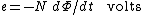for a coil of N turns wound sufficiently closely to make the number of lines linking each the same. The International System (SI) unit of &PHgr;, the weber (Wb), is defined accordingly as the volt-second. The symbol B is used to denote the flux, or line, density, as in Eq. (2),
(2)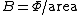when the area of the coil is sufficiently small to sample conditions at a point, and the coil is oriented so that the induced emf is a maximum. The SI unit of B, the tesla (T), is the Wb/m2. The sign of the emf, e, is measured positively in the direction of a right-hand screw pointing in the direction of the flux lines. It is often convenient, particularly when calculating induced emfs, to describe the field in terms of a magnetic vector potential function instead of flux.

#### Magnetic circuits

The magnetic circuit provides a useful method of analyzing devices with ferromagnetic parts, and introduces various quantities used in magnetism. It describes the use of ferromagnetic materials to control the flux paths in a manner analogous to the role of conductors in carrying currents around electrical circuits. For example, pieces of iron may be used to guide the flux which is produced by a magnet along a path which includes an air gap (Fig. 2), giving an increase in the flux density, B, if the cross-sectional area of the gap is less than that of the magnet. See Magnet, Magnetic materials

The magnet may be replaced by a coil of N turns carrying a current, i, wound over a piece of iron, or ferromagnetic material, in the form of a ring of uniform cross section. The flux linking each turn of the coil, and each turn of a secondary coil wound separately from the first, is then approximately the same, giving the same induced emf per turn [according to Eq. (1)] when the supply current, i, and hence the flux, &PHgr;, changes in time. The arrangement is typical of many different devices. It provides, for example, an electrical transformer whose input and output voltages are directly proportional to the numbers of turns in the windings. Emf's also appear within the iron, and tend to produce circulating currents and losses. These are commonly reduced by dividing the material into thin laminations. See Eddy current

The amount of flux produced by a given supply current is reduced by the presence of any air gaps which may be introduced to contribute constructional convenience or to allow a part to move. The effects of the gaps, and of different magnetic materials, can be predicted by utilizing the analogy between flux, &PHgr;, and the flow of electric current through a circuit consisting of resistors connected in series (Fig. 3). Since &PHgr; depends on the product, iN, of the winding current and number

(3)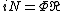of turns, as in Eq. (3), the ratio between them, termed the reluctance, , is the analog of electrical resistance. It may be constant or may vary with &PHgr;. The quantity iN is the magnetomotive force (mmf), analogous to voltage or emf in the equivalent electrical circuit. The relationship between the two exchanges the potental and flow quantities, since the magnetic mmf depends on current, i, and the electrical emf on d&PHgr;/dt. Electric and magnetic equivalent circuits are referred to as duals. See Reluctance

Any part of the magnetic circuit of length l, in which the cross section, a, and flux density, B, are uniform has a reluctance given by Eq. (4). This equation parallels Eq. (5)

(4)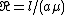(5)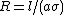for the resistance, R, of a conduct of the same dimensions. The permeability, μ, is the magnetic equivalent of the conductivity, &sgr;, of the conducting material. Using a magnet as a flux source (Fig. 2) gives an mmf which varies with the air gap reluctance. In the absence of any magnetizable materials, as in the air gaps, the permeability is given by Eq. (6)
(6)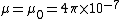in SI units (Wb/A-m). The quantity μ0 is sometimes referred to as the permeability of free space. Material properties are described by the relative permeability, μr in accordance with Eq. (7).
(7)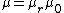The materials which are important in magnetic circuits are the ferromagnetics and ferrites characterized by large value of μr, sometimes in excess of 10,000 at low flux densities.

#### Magnetic field strength

It is convenient to introduce two different measures of the magnetic field: the flux density, B, and the field strength, or field intensity, H. The field strength, H, can be defined as the mmf per meter. It provides a measure of the currents and other magnetic field sources, excluding those representing polarizable materials. It may also be defined in terms of the force on a unit pole.

A straight wire carrying a current I sets up a field (Fig. 4) whose intensity at a point at distance r is given by Eq. (8).

(8)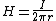The field strength, H, like B, is a vector quantity pointing in the direction of rotation of a right-hand screw advancing in the direction of current flow. The intensity of the field is shown by the number of field lines intersecting a unit area. The straight wire provides one example of the circuital law, known as Ampère's law, given by Eq. (9).
(9)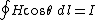Here, Θ is the angle between H and the element dl of any closed path of summation, or integration, and I is the current which links this path. Choosing a circular path, centered on a straight wire, reduces the integral to H (2&pgr;r).

A long, straight, uniformly wound coil (Fig. 5), for example, produces a field which is uniform in the interior and zero outside. The interior magnetic field, H, points in the direction parallel to the coil axis. Applying Eq. (14) to the rectangle pqrs of unit length in the axial direction shows that the only contribution is from pq, giving Eq. (10),

(10)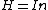where n is the number of turns, per unit length, carrying the current, I. The magnetic field strength, H, remains the same, by definition, whether the interior of the coil is empty or is filled with ferromagnetic material of uniform properties. The interior forms part of a magnetic circuit in which In is the mmf per unit length, where mmf is the magnetic analog of electric voltage, or scalar potential, in an electric circuit. The magnetic field strength, H, is the analog of the electric field vector, E, as a measure of potential gradient, pointing down the gradient. The flux density, B, describes the effect of the field, in the sense of the voltage which is induced in a search coil by changes in time [Eq. (1)]. The ratio of H to B is the reluctance of a volume element of unit length and unit cross section in which the field is uniform, so that, from Eq. (4), the two quantities are related by Eq. (11).
(11)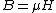The permeability, μ, is defined by Eq. (11). The relative permeability, μr, of polarizable materials is measured accordingly by subjecting a sample to a uniform field inside a long coil such as that shown in Fig. 5 and using the emf induced in a search coil wound around the specimen to observe the flux in it.

#### Magnetic flux and flux density

Magnetic flux is defined in terms of the forces exerted by the magnetic field on electric charge. The forces can be described in terms of changes in flux with time [Eq. (1)], caused either by motion relative to the source or by changes in the source current, describing the effect of charge acceleration.

Since the magnetic, or electrokinetic, energy of current flowing in parallel wires depends on their spacing, the wires are subject to forces tending to change the configuration. The force, dF, on an element of wire carrying a current, i, is given by Eq.

(12)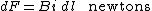(12), and this provides a definition of the flux density, B, due to the wires which exert the force. The SI unit of B, called the tesla, or Wb/m2, is the N/A-m. The flux density, B, equals μ0 H in empty space, or in any material which is not magnetizable [Eq. (11)]. An example is the force, F, per meter (length) which is exerted by a long straight wire on another which is parallel to it, at distance r. From Eq. (8), this force is given by Eq. (13),
(13)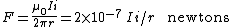when the wires carry currents I and i. The force, F, is accounted for by the electrokinetic interactions between the conduction charges, and describes the relativistic modification of the electric forces between them due to their relative motion.

In general, any charge, q, moving at velocity u is subject to a force given by Eq. (14),

(14)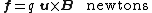where u × B denotes the cross-product between vector quantities. That is, the magnitude of f depends on the sine of the angle Θ between the vectors u and B , of magnitudes u and B, according to Eq. (15).
(15)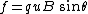The force on a positive charge is at right angles to the plane containing u and B and points in the direction of a right-hand screw turned from u to B .

The same force also acts in the axial direction on the conduction electrons in a wire moving in a magnetic field, and this force generates an emf in the wire. The emf in an element of wire of length dl is greatest when the wire is at right angles to the B vector, and the motion is at right angles to both. The emf is then given by Eq. (16).

(16)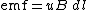More generally, u is the component of velocity normal to B , and the emf depends on the sine of the angle between dl and the plane containing the velocity and the B vectors. The sign is given by the right-hand screw rule, as applied to Eq. (15).

The magnetic flux linking any closed path is obtained by counting the number of flux lines passing through any surface, s, which is bounded by the path. Stated more formally, the linkage

(17)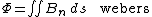depends on the sum given in Eq. (17), where Bn denotes the component of B in the direction normal to the area element, ds. The rate of change of linkage gives the emf induced in any conducting wire which follows the path [Eq. (1)].

The flux linkage with a coil (Fig. 6) is usually calculated by assuming that each turn of the coil closes on itself, giving a flux pattern which likewise consists of a large number of separate closed loops. Each links some of the turns, so that the two cannot be separated without breaking, or “tearing,” either the loop or the turn. The total linkage with the coil is then obtained by adding the contributions from each turn.

The inductance, L, is a property of a circuit defined by the emf which is induced by changes of current in time, as

(18)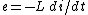in Eq. (18). The SI unit of inductance is the henry (H), or V-s/A. The negative sign shows that e opposes an increase in current (Lenz's law). From Eq. (1) the inductance of a coil of N turns, each linking the same flux, &PHgr;, is given by Eq. (19),
(19)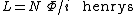so that the henry is also the Wb/A. When different turns, or different parts of a circuit, do not link the same flux, the product N&PHgr; is replaced by the total flux linkage, &PHgr;, with the circuit as a whole.

The mutual inductance, M, between any two coils, or circuit parts, is defined by emf which is induced in one by a change of current in the other. Using 1 and 2 to distinguish between them, the emf induced in coil 1 is given by Eq. (20a),

(20{\em a})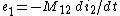(20{\em b})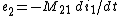where the sign convention is consistent with that used for L, referred to as the self-inductance. Likewise, the emf induced in coil 2 when the roles of the windings are reversed is given by Eq. (20b). The interaction satisfies the reciprocity condition of Eq. (21), so that the suffixes may be omitted.
(21)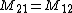#### Magnetostatics

The term “magnetostatics” is usually interpreted as the magnet equivalent of the electrostatic interactions between electric charges. The equivalence is described most directly in terms of the magnetic pole, since the forces between poles, like those between charges, vary inversely with the square of the separation distance. Although no isolated poles, or monopoles, have yet been observed, the forces which act on both magnets and on coils are consistent with the assumption that the end surfaces are equivalent to magnetic poles.

#### Magnetic moment

The magnetic moment of a small current loop, or magnet, can be defined in terms of the torque which acts on it when placed in a magnetic flux density, B, which is sufficiently uniform in the region of the loop. For a rectangular loop with dimensions a and b and with N turns, carrying a current, i, equal but opposite forces act on the opposite sides of length a (Fig. 7). The force is iNBa [Eq. (12)], and the torque, given by

(22)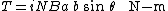Eq. (22), depends on the effective distance, b sin Θ, between the wires. It is proportional to the area ab, and is a maximum when the angle Θ between B and the axis of the loop is 90°. A current loop of any other shape can be replaced by a set of smaller rectangles placed edge to edge, and the torques of these added to give the total on the loop. The magnetic moment of any loop of area s is defined as the ratio of the maximum torque to the flux density, so
(23)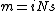its magnitude is given by Eq. 23). It is a vector quantity pointing in the direction of a right-hand screw turned in the direction of current flow. It is expressed in vector cross-product notation by Eq. (24).
(24)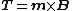See Torque

An electron orbiting at frequency f is the equivalent of a current i = q f, giving

(25)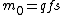Eq. (25) for the moment, where s is the area of the orbit. The permissible values are determined by the quantum energy levels. The electron spin is a quantum state which can likewise be visualized as a small current loop. Atomic nuclei also possess magnetic moments. See Electron spin, Magneton, Nuclear moments

#### Magnetic polarization

Materials are described as magnetic when their response to the magnetic field controls the ratio of B to H. The behavior is accounted for by the magnetic moments produced mainly by the electron spins and orbital motions. These respond to the field and contribute to it in a process referred to as magnetic polarization. The effects are greatest in ferromagnetics and in ferrites, in which the action is described as ferrimagnetic. See Ferrimagnetism, Ferromagnetism

The sources are the equivalent of miniature “Ampèrean currents” whose sum, in any volume element, is equivalent to a loop of current flowing along the surface of the element. The flux density, B, depends on the field intensity, H, which is defined so that its value inside a long ferromagnetic rod of uniform cross section placed inside a long coil (Fig. 5) is the same as in the annular gap between the rod and the coil, in accordance with Eq. (10). If the field is not sufficiently uniform, H can be measured by using a search coil to observe the flux density, μ0 H, in the gap. The flux density inside the rod is given by Eq. (26),

(26)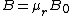where B0 denotes μ0H, and μr is the relative permeability [Eqs. (7) and (11)]. The same flux, B, is obtained by replacing the material by a coil in which the current in amperes per unit length
(27)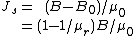is given by Eq. (27). The magnetic moment, dm, of a volume element of length dz is due to the current flowing over the surface enclosing the area, dydz; from Eq. (23), it is given by Eq. (28). The moment per unit volume defines the magnetic polarization, as
(28)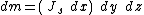(29)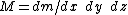in Eq. (29). The polarization, M , is a vector pointing in the direction of dm with magnitude Js. The surface current produces an H -like, or B0, field which is entirely different from H in the material. Substituting from Eq. (27) gives Eq. (30).
(30)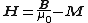This model of the material accounts for the flux field, B , as observed by the voltage induced in a search coil wound around the specimen, and H , becomes an auxiliary quantity representing the sum of the polarization, M , and the magnetizing field, B μ0, to which M responds. The polarization, M , also makes the largest contribution to that field, since the equivalent surface current is in the same direction as the current in the magnetizing coil.

#### Magnetic hysteresis

The relationship between the flux density, B, and the field intensity, H, in ferromagnetic materials depends on the past history of magnetization. The effect is known as hysteresis. It is demonstrated by subjecting the material to a symmetrical cycle of change during which H is varied continuously between the positive and negative limits +Hm and -Hm (Fig. 8). The path that is traced by repeating the cycle a sufficient number of times is the hysteresis loop. The sequence is counterclockwise, so that B is larger when H is diminishing than when it is increasing, in the region of positive H. The flux density, Br, which is left when H falls to zero is called the remanence, or retentivity. The magnetically “hard” materials used for permanent magnets are characterised by a high Br, together with a high value of the field strength, -Hc, which is needed to reduce B to zero. The field strength, Hc, is known as the coercive force, or coercivity. Cycling the material over a reduced range in H gives the path in Fig. 8 traced by the broken line, lying inside the larger loop. The locus of the tips of such loops is known as the normal magnetization curve. The initial magnetization curve is the B-H relationship which is followed when H is progressively increased in one direction after the material has first been demagnetized (B = H = 0).

McGraw-Hill Concise Encyclopedia of Physics. © 2002 by The McGraw-Hill Companies, Inc.
The following article is from The Great Soviet Encyclopedia (1979). It might be outdated or ideologically biased.

## Magnetism

a phenomenon manifested on a macroscopic scale as interaction between electric currents, between currents and magnets (that is, bodies having a magnetic moment), and between magnets. In its most general form, magnetism may be defined as a special type of the physical interactions that arise between moving electrically charged particles. The transfer of magnetic interaction, which forms a bond between spatially separated bodies, is accomplished by a special material carrier, the magnetic field. It, together with the electric field, is a manifestation of the electromagnetic form of the motion of matter. Full symmetry between the magnetic and electric fields does not exist. The sources of the electric field are the electric charges of elementary particles, such as electrons, protons, and mesons. Analogous magnetic charges have not yet been observed in nature, although hypotheses of their existence have been set forth.

A moving electric charge—that is, an electric current—is the source of a magnetic field. On the atomic scale there are two types of microscopic currents for electrons and nucleons (protons and neutrons): orbital currents, which are associated with the translatory motion of the center of gravity of the particles, and spin currents, which are associated with the internal degrees of freedom of their motion.

The orbital and spin magnetic moments of particles (designated as M) are the quantitative characteristic of their magnetism. Since all microscopic structural elements of matter (electrons, protons, and neutrons) have magnetic moments, any combinations of them (atomic nuclei and electron shells) and combinations of their combinations, or atoms, molecules, and macroscopic bodies, may in principle be magnetic sources. Thus, the magnetism of substances is universal.

Two basic effects of the action of an external magnetic field on substances are known. The first is the diamagnetic effect, which is a consequence of Faraday’s law of electromagnetic induction: an external magnetic field always creates in a substance an induction current whose magnetic field is directed against the original field (Lenz’s law). Therefore, the diamagnetic moment of a substance that is generated by an external field is always negative with respect to the field.

Second, if an atom has a nonzero magnetic moment (spin or orbital moment, or both), an external field will tend to orient it along its own direction. A positive moment that is parallel to the field, called the paramagnetic moment, arises as a result.

Internal interactions of an electrical and magnetic nature between atomic magnetic moments may also significantly influence the magnetic properties of a substance. In some cases, as a result of these interactions the existence in the substance of a spontaneous atomic magnetic order that is independent of the external field becomes more advantageous in terms of energy. Substances in which the atomic magnetic moments are parallel to each other are called ferromagnets; substances in which neighboring atomic moments are antiparallel are called antiferromagnets. The complexity of the atomic structure of substances constructed from an extremely large number of atoms leads to the virtually inexhaustible diversity of their magnetic properties. The general term “magnets” is used in examining the magnetic properties of substances. The interrelation between the magnetic properties of substances and their nonmagnetic properties (such as electrical, mechanical, and optical properties) very often makes possible the use of research on magnetic properties as a source of information on the internal structure of microscopic particles and macroscopic bodies. As a result of the broad range of magnetic phenomena, which extends from the magnetism of elementary particles to the magnetism of celestial objects (such as the earth, sun, and stars), magnetism plays a major role in natural phenomena, science, and technology.

The macroscopic description of the magnetic properties of substances is usually given within the framework of electromagnetic field theory, thermodynamics, and statistical physics. The magnetization vector J (the total magnetic moment per unit volume of a magnet) is one of the principal macroscopic characteristics of a magnet that determine its thermodynamic state. Experiments show that the vector J is a function of the magnetic field intensity H. The relation J(H) is represented graphically by the magnetization curve, which has a different form for different magnets. The linear relation J = KH, where K is the magnetic susceptibility (in diamagnets K < 0; in paramagnets K > 0), exists in a number of substances. In ferromagnets K has a nonlinear relation to H; for them the susceptibility is dependent not only on the temperature T and the properties of the substance but also on the field H.

The magnetization J of a magnet is defined thermodynamically in terms of the thermodynamic potential Φ = (H, T, p ) according to the formula J = -(∂Φ/∂H)T,P, where ρ is the pressure. The calculation of Φ (H, T, p ), in turn, is based on the Gibbs-Boguslavskii equation Φ = —kT ln Z(H, T) where k is the Boltzmann constant and Z(H, T) is the statistical sum.

It follows from the general postulates of classical statistical physics that electron systems (disregarding their quantum properties) cannot have a thermodynamically stable magnetic moment (the Bohr-Van Leeuwen-Terletskii theorem), but this contradicts experiment. Quantum mechanics, which explained the stability of the atom, also gave an explanation of the magnetism of atoms and macroscopic bodies.

The magnetism of atoms and molecules results from the spin magnetic moments of their electrons, the motion of electrons in the shells of atoms and molecules (the orbital magnetism), and the spin and orbital magnetism of the nucleons. In many-electron atoms the orbital and spin magnetic moments are added according to the rules of spatial quantization: the resultant magnetic moment is determined by the total angular quantum number j and is equal to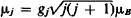where gj is the Lande factor and μB is the Bohr magneton.

The magnetic properties of substances are determined by the nature of the atomic carriers of magnetism and by the character of their interactions. In particular, comparison of the magnetic properties of isolated atoms of various elements indicates the significant influence exerted by these interactions on magnetic properties. For example, in atoms of the inert gases, such as helium, argon, and neon, the electron shells are magnetically neutral (their total magnetic moment is equal to zero). In an external magnetic field inert gases exhibit diamagnetic properties. The electron shell of the atoms of alkali metals (such as lithium, sodium, and potassium) have only the spin magnetic moment of the valence electron, and the orbital moment of such atoms is equal to zero. The vapor of an alkali metal is paramagnetic. Large spin and orbital magnetic moments caused by the unfilled d- and f-layers of their electron cloud are usually observed in the atoms of the transition metals (such as iron, cobalt, and nickel).

The strong dependence of the magnetism of substances on the character of the bonds among microscopic particles (the carriers of magnetic moment) accounts for the fact that a substance of given chemical composition may have different magnetic properties, depending on the external conditions and the crystal or phase structure (such as the degree of order of the atoms in alloys). For example, below a certain temperature (the Curie point) iron, cobalt, and nickel in the crystalline state have ferro-magnetic properties. Above the Curie point they lose those properties.

In quantitative terms the interaction between the atomic carriers of magnetism in a substance may be characterized by the amount of energy €int of the interaction, referred to a pair of particles that are carriers of magnetic moment. The energy €int, which results from the electric and magnetic interaction of microscopic particles and depends on their magnetic moments, may be compared with the energy values of other atomic interactions, such as the energy of the magnetic moment μB in some effective magnetic field Heff (that is, with €H = μBHeff), and the average energy of thermal motion of the particles at some effective critical temperature Tc (that is, with €T = kTc). For values of the external field intensity H < Heff or at temperatures T < Tc, the magnetic properties of a substance caused by ∈int—the internal interactions of the atomic carriers of magnetism (the “strong” magnetism of substances)—will be manifested strongly. Conversely, external factors such as the temperature or field, which suppress the effect of internal interaction (the “weak” magnetism of substances), will dominate in regions where H » Heff or T » Tc. This classification is formal, since it does not reveal the physical nature of Heff and Tc.

For a full explanation of the physical nature of the magnetic properties of a substance, it is necessary to know not only the amount of energy €int in comparison with TOr €H but also its physical origin and the nature of the magnetic moment of its carriers (whether of the orbital or spin type). If the case of nuclear magnetism (in which the effect of nuclear interactions is manifested) is excluded, then two types of forces—electric and magnetic—operate in the electron shells of atoms and molecules and in the electron systems of condensed substances (liquids or crystals). The electrostatic energy €e1 of two electrons separated by the atomic distance (a = 10-8 cm), €el ~ e2/a ~ 10-12 erg (where e is the charge of an electron), may be a measure of electrical interaction. The binding energy of two microscopic particles with magnetic moments μB, separated by a distance a (that is, €mag ˜ μB2/a3 ~ 1016 erg), is the measure of magnetic interaction. Thus, €el exceeds the energy €mag by at least three orders of magnitude.

In connection with this, the retention of magnetization by ferromagnets (Fe, Co, and Ni) to temperatures T ~ 1000°K may be due solely to electrical interaction, since at an energy €mag ~ 1016 erg thermal motion would destroy the orienting action of magnetic forces even at 1°K. On the basis of quantum mechanics, it has been demonstrated that a purely quantum electrostatic exchange interaction that depends on the relative orientation of the magnetic moments of electrons exists, in addition to the Coulomb electrostatic interaction of charged particles. Thus, this part of an interaction that is electric in nature has a significant effect on the magnetic state of electron systems. In particular, the interaction promotes the ordered orientation of the magnetic moments of the atomic carriers of magnetism. The upper limit of the energy of the exchange interaction is €ex ~ 10-13 erg.

A value ∈ex > 0 corresponds to parallel orientation of atomic magnetic moments—that is, to spontaneous magnetization of the bodies (ferromagnets). When €ex < 0 there is a tendency toward antiparallel orientation of neighboring magnetic moments that is characteristic of the atomic magnetic structure of antiferromagnets. This makes possible the following physical classification of the magnetism of substances.

Magnetism of weakly interacting particles (€int « μBH or €int « kT). PREDOMINANCE OF DIAMAGNETISM. Among the substances that have diamagnetic properties are the following.

(1) All inert gases, as well as gases whose atoms or molecules do not have an intrinsic net magnetic moment. Their magnetic susceptibility is negative and very small in magnitude [molar susceptibility x ~ — (10-7-10-5)]; it is virtually independent of temperature.

(2) Organic compounds with a nonpolar bond, in which the molecules or radicals either have no magnetic moment or the paramagnetic effect is suppressed by the diamagnetic effect. In such compounds x ~ -10-6 and also is virtually independent of temperature but does have noticeable anisotropy.

(3) Substances in condensed (liquid or crystalline) phases—for example, certain metals (such as zinc, gold, and mercury), solutions, alloys, and chemical compounds (such as the halides)—in which the diamagnetism of ion cores (ions similar to the atoms of inert gases such as Li+, Be2+, Al3+ , and Cl-) predominates. The magnetism of this group of substances is similar to the magnetism of “classical” diamagnetic gases.

PREDOMINANCE OF PARAMAGNETISM. Predominance of paramagnetism is characteristic of the following groups.

(1) Free atoms, ions, and molecules that have a net magnetic moment. The gases O2 and NO and the vapors of the transition and alkali metals are paramagnetic. Their susceptibility, x > 0, is small in magnitude (~ 10 3-10-5) and, at temperatures that are not very low and in magnetic fields that are not very strong (μBH/kT « 1), is independent of the field but strongly dependent on the temperature. Here Curie’s law, x = C/T, is valid for x, where C is the Curie constant.

(2) Ions of the transition elements in liquid solutions, and also in crystals, provided that the magnetically active ions interact weakly with each other and that their immediate environment in the condensed phase has a weak influence on their paramagnetism. When μBH/KT « 1, the susceptibility x is independent of H but dependent on T, and the Curie-Weiss law, x = C/(T - Δ), is valid, where C and Δ are constants of the substance.

(3) Ferromagnets and antiferromagnets above the Curie point Θ.

Magnetism of conduction electrons in metals and semiconductors. PARAMAGNETISM OF CONDUCTION ELECTRONS IN METALS. Paramagnetism of conduction electrons in metals (spin paramagnetism) is observed in the alkali metals (such as lithium, potassium, and sodium), the alkaline earths (calcium, strontium, barium, and radium), the transition metals (palladium and platinum), and scandium, titanium, and vanadium. Their susceptibility is low (x ~ 10-5) and independent of field, and it changes slightly with temperature. In several metals (such as copper, silver, and gold), the paramagnetism is concealed by the stronger diamagnetism of the ion cores.

DIAMAGNETISM OF CONDUCTION ELECTRONS IN METALS. Diamagnetism of conduction electrons in metals (Landau diamagnetism) is inherent in all metals but is usually concealed by stronger spin electron paramagnetism or by the diamagnetism or paramagnetism of the ion cores.

PARAMAGNETISM AND DIAMAGNETISM OF CONDUCTION ELECTRONS IN SEMICONDUCTORS. In comparison with metals, semiconductors have few conduction electrons, but their number increases with temperature; in this case x is also dependent on T.

MAGNETISM OF SUPERCONDUCTORS. The magnetism of superconductors is due to electric currents flowing in a surface layer with a thickness of the order of 10-5 cm. The currents shield the body of the superconductor from external magnetic fields; therefore, in a massive superconductor the magnetic field is equal to zero when T < Tc (the Meissner effect).

Magnetism of substances with atomic magnetic order (€int » μB or €int » kT). FERROMAGNETISM. Ferromagnetism exists in substances that have a positive exchange energy (€ex > 0): crystals of iron, cobalt, and nickel and a number of rare earths (gadolinium, terbium, dysprosium, holmium, erbium, thulium, and ytterbium) and alloys and compounds of these elements, as well as alloys of chromium and manganese and compounds of uranium. Spontaneous magnetization at temperatures below the Curie point Θ is characteristic of ferromagnetism, and when T > Θ ferromagnets pass into the paramagnetic or antiferromagnetic state (the latter case is observed, for example, in some rare earths). However, experiments have shown that ferromagnetic solids have no net magnetization in the absence of an external field (if the secondary phenomenon of residual magnetization is disregarded); this is because when H = 0 a ferromagnet breaks down into a large number of microscopic, spontaneously magnetized regions (domains). The magnetization vectors of the individual domains are oriented in such a way that the total magnetization of the ferromagnet is equal to zero. In an external field the domain structure changes, and the ferromagnet acquires net magnetization.

ANTIFERROMAGNETISM. Antiferromagnetism exists in substances that have a negative exchange energy (€ex < 0): crystals of chromium and manganese, a number of rare earths (cesium, praseodymium, neodymium, samarium, and europium), and numerous compounds and alloys in which elements of transition groups are found. In magnetic terms the crystal lattice of such substances breaks down into magnetic sublattices, whose spontaneous magnetization vectors Jki, are either antiparallel (collinear antiferromagnetic bond) or are opposed to each other at angles that differ from 0° and 180° (noncollinear bond). If the total moment of all magnetic sublattices in an antiferromagnet is equal to zero, then compensated antiferromagnetism is present, but uncompensated antiferromagnetism, or ferrimagnetism, which is found chiefly in crystals of metal oxides with a crystal lattice of the spinel type, such as garnet and perovskite (called ferrites), is observed in the case of nonzero differential spontaneous magnetization. These solids (usually semiconductors and insulators) are similar in magnetic properties to ordinary ferromagnets. When the compensation for magnetic moments is disturbed in antiferromagnets because of the weak interaction between the atomic carriers of magnetism, very slight spontaneous magnetization of the substances takes place (of the order of 0.1 percent of the ordinary values for ferromagnets and ferrimagnets). Such substances are called weak ferromagnets; examples are hematite, a-Fe2O3, and the carbonates of a number of metals and orthoferrites.

The magnetic state of a ferromagnet or antiferromagnet in an external magnetic field H is determined by the previous state of the magnet (the magnetic prehistory of the sample), in addition to the field strength. This phenomenon is called hysteresis. Magnetic hysteresis is manifested in the indeterminacy of the dependence of J on H (in the presence of a hysteresis loop). Because of hysteresis, elimination of the external field is insufficient for demagnetization of a specimen, since when H = 0 the specimen will retain its residual magnetization Jr. An opposite magnetic field Hc, which is called the coercive force, must be applied. A distinction is made between soft-magnetic materials (Hc < 800 amperes per meter [A/m], or 10 oersteds) and hard-magnetic, or high-coercivity, materials (Hc > 4 kA/m, or 50 oersteds), depending on the value of Hc. The quantities Jr and Hc are dependent on temperature and generally decline as the temperature increases, tending toward zero as T approaches Θ.

In addition to the magnetism of atomic particles and substances, the current study of magnetic phenomena includes the magnetism of celestial bodies and outer space.

Magnetism in science and technology. Among the primary problems of the current study of magnetism are the elucidation of the nature of exchange interaction and the interactions responsible for anisotropy in various types of magnetically ordered crystals and the determination of the spectra of elementary magnetic excitations (magnons) and of the mechanisms of their interaction with each other and with phonons (quanta of lattice vibrations). The development of a theory of the transition from the paramagnetic state to the ferromagnetic state remains an important problem. Research on the magnetism of substances is used extensively in various fields of science as a means of studying the chemical bonds and structure of molecules (magneto-chemistry). The study of the diamagnetic and paramagnetic properties of gases, liquids, solutions, and compounds in the solid phase makes possible an understanding of the details of the physical and chemical processes that take place within the substances, and also of their structure. The study of magnetic dynamic characteristics (paramagnetic, diamagnetic, and ferromagnetic resonance; electron and nuclear resonances and relaxations) aids in understanding the kinetics of many physical and physicochemical processes in various substances. Magnetobiology is developing rapidly.

The elucidation of the origin of the magnetic fields of the earth, planets, sun, stars (in particular pulsars), and extragalactic radio sources (such as radio galaxies and quasars) and of the role of magnetic fields in cosmic processes are among the most important problems of the magnetism of cosmic bodies.

The main technical applications of magnetism are in electrical engineering, radio engineering, electronics, instrument-making, electronic computers, and maritime, air, and space navigation, as well as geophysical methods of prospecting and automatic and remote-control equipment. Magnetic flaw detection and magnetic inspection techniques are also widely used. Magnetic materials are used to manufacture the magnetic circuits of generators, motors, transformers, relays, magnetic amplifiers, magnetic memory components, compass pointers, and magnetic tapes.

History of the study of magnetism. The first written references to magnetism (from China) are more than 2,000 years old. The use of natural permanent magnets as compasses is mentioned in the references. The attraction and repulsion of natural magnets and magnetization of iron filings in the presence of a magnet are mentioned in works of ancient Greek and Roman scholars (for example, in Lucretius’ narrative poem On the Nature of Things, first century B.C.). In the Middle Ages (beginning in the 12th century) the magnetic compass became widely used in Europe, and attempts at the experimental study of the interaction of magnets of different shapes were made (Petrus Peregrinus de Maricourt, 1269). The results of studies of magnetism during the Renaissance were summarized in W. Gilbert’s work On the Magnet, Magnetic Bodies, and a Large Magnet, the Earth (1600). In particular, Gilbert showed that the earth is a magnetic dipole and proved the impossibility of separating the two poles of a magnet. The study of magnetism was further developed in the works of R. Descartes, F. Aepinus, and C. Coulomb. Descartes originated the first detailed metaphysical theory of magnetism and geomagnetism (Principles of Philosophy, part 4, 1644); he postulated the existence of a special magnetic substance that by its presence and motion accounted for the magnetism of bodies.

In the treatise Experiment in the Theory of Electricity and Magnetism (1759), Aepinus underscored the close analogy between electrical and magnetic phenomena. As Coulomb showed (1785-89), the analogy has a specific quantitative expression: the interaction of point magnetic poles conforms to the same law as does the interaction of point electric charges (Coulomb’s law). In 1820, C. Oersted discovered the magnetic field of an electric current. In the same year A. Ampere established the laws governing the magnetic interaction of currents and the equivalence of the magnetic properties of a ring current and a thin flat magnet; he explained magnetism in terms of the existence of molecular currents. In the 1830’s, K. Gauss and W. Weber developed the mathematical theory of geomagnetism and worked out methods of magnetic measurements.

A new stage in the study of magnetism began with the work of M. Faraday, who gave a consistent interpretation of magnetic phenomena on the basis of his ideas that the electromagnetic field actually exists. The groundwork for the modern macroscopic theory of magnetism was laid by a number of the most important discoveries in the field of electromagnetism (electromagnetic induction, Faraday, 1831; Lenz’s law, E. H. Lenz, 1833), by the summary of known electromagnetic phenomena in the works of J. C. Maxwell (1872), and by the systematic study of the properties of ferromagnets and paramagnets (A. G. Stoletov, 1872; P. Curie, 1895).

The microscopic approach to the study of magnetism became possible after the discovery of the electron-nuclear structure of atoms. In 1905, P. Langevin constructed, on the basis of H. A. Lorentz’s classical electron theory, the theory of diamagnetism (he also developed the quasiclassical theory of paramagnetism). B. L. Rozing in 1892 and P. Weiss in 1907 expressed the idea of the existence of an internal molecular field that accounts for the properties of ferromagnets. The discovery of electron spin and its magnetism (S. Goudsmit and G. E. Uhlenbeck, 1925) and the creation of a consistent theory of microscopic phenomena— quantum mechanics—led to the development of the quantum theory of diamagnetism, paramagnetism, and ferromagnetism. In 1926, on the basis of quantum mechanical concepts (space quantization), L. Brillouin found the dependence of the magnetization of paramagnets on an external magnetic field and temperature. In 1927, F. Hund made a comparison of the experimental and theoretical values of the effective magnetic moments of ions in various paramagnetic salts. This led to elucidation of the influence of the electric fields of a paramagnetic crystal on the “quenching” of the orbital angular moments of ions—as was established, the magnetization of a crystal is determined almost exclusively by spin moments (W. G. Penney and R. Schlapp; J. H. Van Vleck, 1932). In the 1930’s the quantum mechanical theory of the magnetic properties of free electrons was constructed (Pauli paramagnetism, 1927; Landau diamagnetism, 1930). The phenomenon of electron paramagnetic resonance (EPR), predicted by Ia. G. Dorfman (1923) and later discovered by E. K. Zavoiskii (1944), was of great significance to the further development of the theory of paramagnetism.

The works of the German physicist E. Ising (1925; the two-dimensional model of ferromagnets) and of Dorfman (1927; proof of the nonmagnetic nature of the molecular field), W. Heisenberg (1926; the quantum mechanical calculation of the helium atom), and W. Heitler and F. London (1927; calculation of the hydrogen molecule) preceded the creation of the quantum theory of ferromagnetism. In the last two works the effect of the exchange (electrostatic) interaction of electrons (P. Dirac, 1926) in the shells of atoms and molecules, which was discovered in quantum mechanics, was used and its relation to the magnetic properties of electron systems that obey Fermi-Dirac statistics (the Pauli exclusion principle) was established. The quantum theory of ferromagnetism originated in the works of Ia. I. Frenkel’ (1928; the collectivized model) and Heisenberg (1928; the model of localized spins). Consideration of ferromagnetism as a quantum cooperative phenomenon (F. Bloch and J. Slater, 1930) led to the discovery of spin waves. In 1932-33, L. Neel and L. D. Landau predicted the existence of antiferromagnetism. This study of the new classes of magnetic substances —antiferromagnets and ferrites—made possible a more thorough understanding of the nature of magnetism. The role of magnetoelastic energy in the origin of the energy of magnetic anisotropy was elucidated, the theory of domain structure was constructed, and the methods for its experimental study were mastered.

The creation of new experimental methods of studying substances contributed greatly to the development of magnetism. Neutron-diffraction methods made possible determination of the types of atomic magnetic structures. Ferromagnetic resonance, which was originally discovered and investigated in the works of V. K. Arkad’ev (1913) and later J. H. E. Griffiths (1946), and antiferromagnetic resonance (C. J. Gorter and others, 1951) made possible the beginning of experimental studies of the processes of magnetic relaxation and provided an independent method of determining the effective fields of anisotropy in ferromagnets and antiferromagnets.

Nuclear magnetic resonance (E. Purcell and others, 1946) and the Mössbauer effect (1958) greatly extended knowledge of the distribution of spin density in matter, especially in metal ferromagnets> Observation of neutron and light scattering made possible determination of the spin-wave spectra for a number of substances. Simultaneously with these experimental works various aspects of the theory of magnetism also were developed: the theory of the magnetic symmetry of crystals, the ferromagnetism of collectivized electrons, the theory of second-order phase transitions and critical phenomena, and models of one- and two-dimensional ferromagnets and antiferromagnets.

The development of the physics of magnetic phenomena has led to the synthesis of promising new magnetic materials: ferrites for high-frequency and superhigh-frequency devices, high-coercivity compounds such as SmCos, and transparent ferromagnets.

### REFERENCES

Tamm, I. E. Osnovy teorii elektrichestva, 7th ed. Moscow, 1957.
Landau, L. D., and E. M. Lifshits. Elektrodinamika sploshnykh sred. Moscow, 1959.
Vonsovskii, S. V. Magnetizm. Moscow, 1971.
Peierls, R. E. Kvantovaia teoriia tverdykh tel Moscow, 1956. (Translated from English.)
Dorfman, Ia. G. Magnitnye svoistva i stroenie veshchestva. Moscow, 1955.
Kittel, C. Vvedenie v fiziku tverdogo tela, 2nd ed. Moscow, 1962. (Translated from English.)
Vonsovskii, S. V., and Ia. S. Shur. Ferromagnetizm. Moscow-Leningrad, 1948.
Polivanov, K. M. Ferromagnetiki. Moscow-Leningrad, 1957.
Bozorth, R. Ferromagnetizm. Moscow, 1956. (Translated from English.)
Mattis, D. Teoriia magnetizma: Vvedenie v izuchenie kooperativnykh iavlenii. Moscow, 1967. (Translated from English.)
Turov, E. A. Fizicheskie svoistva magnitouporiadochennykh kristallov. Moscow, 1963.
Van Vleck, J. H. The Theory of Electric and Magnetic Susceptibilities. Oxford, 1932.
Becker, R., and W. Doring. Ferromagnetismus. Berlin, 1939. Magnetism, vol. 1; vol. 2, parts A-B; vol. 3; vol. 4. Edited by G. T. Rado and H. Suhl. New York, 1963-66.
Goodenough, J. Magnetism and the Chemical Bond. New York-London, 1963.

S. V. VONSOVSKII

## magnetism

[′mag·nə‚tiz·əm]
(physics)
Phenomena involving magnetic fields and their effects upon materials.
McGraw-Hill Dictionary of Scientific & Technical Terms, 6E, Copyright © 2003 by The McGraw-Hill Companies, Inc.

## magnetism

1. the property of attraction displayed by magnets
2. any of a class of phenomena in which a field of force is caused by a moving electric charge
3. the branch of physics concerned with magnetic phenomena
Collins Discovery Encyclopedia, 1st edition © HarperCollins Publishers 2005
References in periodicals archive ?
(37) He went on to connect "Zoomagnetism" with the sword swallowing of an "Indian conjurer." (38) The magnetist's mysterious apparatus--his baton and his tub--were, presumably, equivalent to the Indians' bayonet.
William Pitt was not just a wizard but also an animal magnetist because he possessed this power.
In the magnetist's Parisian and London theaters of bodily desire and complicity, Coleridge saw scenes that he was able to restage in his narrative poems.
And so there arose a new generation of magnetists for whom the Commission's verdicts were more a stimulus than an embarrassment.
Despite the limitations on its international marketability, Lense-Moller says she prefers shooting "Magnetist" in Norwegian rather than English.
Like virtually every Norwegian production, "Magnetist" lacks a homegrown star or director who can cross over to the international market and thus attract global interest to the country's cinema, much as Ingmar Bergman did for Sweden.
Magic Hour will follow "Magnetist" with another feature, the Polish / Danish "Who Was David Weiser?", based on a prize-winning novel by Pawel Huelle.

Site: Follow: Share:
Open / Close